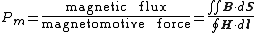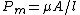# permeance

(redirected from permeances)
Also found in: Dictionary.

## Permeance

The reciprocal of reluctance in a magnetic circuit. It is the analog of conductance (the reciprocal of resistance) in an electric circuit, and is given by

(1)Eq. (1), where B is the magnetic flux density, H is the magnetic field strength, and the integrals are respectively over a cross section of the circuit and around a path within it.

From Eq. (1), it can be shown that Eq. (2) is valid, where A

(2)is the cross-sectional area of the magnetic circuit, l its length, and μ the permeability. If the material is ferromagnetic, as is often the case, then μ is not constant but varies with the flux density and the complete magnetization curve of B against H may have to be used to determine the permeance. See Reluctance

## permeance

[′pər·mē·əns]
(electromagnetism)
A characteristic of a portion of a magnetic circuit, equal to magnetic flux divided by magnetomotive force; the reciprocal of reluctance. Symbolized P.

## permeance

A measure of a material’s resistance to water-vapor transmission, expressed in perms. Equal to the ratio of (a) the rate of water vapor transmission through a material or assembly between its two parallel surfaces to (b) the vapor pressure differential between the surfaces.
Mentioned in ?
References in periodicals archive ?
The determination of the magnetic circuit parameters for the considered machine begins with defining the permeance of the magnet [P.
The selectivity up to 45 and the permeance up to 0.
The gas permeance of the membranes was measured by soap film flow meter under the feed pressure of 1-4 kg/[cm.
2006) have reported that vapour permeabilities or permeances apparently depend on both temperature and feed composition of vapour--permanent mixtures for vapour-selective membrane materials.
Once all the permeances are computed, the sum of these permeances (in webers per ampere) can be obtained.
An air-impermeable material has an air permeance equal to or less than 0.
4] permeances increased significantly for casting solution including higher concentration of BuOH (Fig.
2] permeances of gas mixture are significantly higher than those of pure gas at similar operating conditions.
Reference  has presented the design, optimization of an Outer Rotor OR-PMSM based on coupling boundary element method with permeances network.
When inorganic nanoparticles are added to the polymeric phase, it is predictable that resulting membrane separation properties become better than customary polymeric membranes due to the high gas permeance and selectivity [8-10].

Site: Follow: Share:
Open / Close